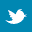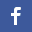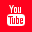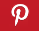Completely Solved C, C++ Programs Assignment.Quick Search Database Videos Tutorials Ebooks Forums FAQ Aboutus Household Industrial Manufacturing Service Shopping Transportation### C++ Program to represent fraction with numerator and denominator as integer pointers

 Filed Under: C++ Assignments

Problem Statement: Implement a class FRACTION to represent fractions with numerator and denominator as integer pointers, and appropriate constructor,copy constructor, assignment operator, addition operator, multiplication operator, subtraction operator and division operator as members.

Logical Approach :
The above program is done using an Abstract Data Type (ADT) called class. A class is a mechanism to create objects. In this program a class named fraction is created.
It contains the following data members :
• num(denoting numerator)
• deno(denoting denominator)
It contains the following member functions :
• fraction()-Default constructor.
• fraction(int,int)-Parameterized constructor, used to initialize a fraction.
• fraction(const fraction&)-Copy constructor, used to make a new copy of a fraction.
• printfrac()-Used to display a fraction.
• operator+(fraction)-Used to add two fractions.
• operator-(fraction)-Used to subtract two fractions.
• operator*(fraction)-Used to multiply two fractions.
• operator/(fraction)-Used to divide two fractions.
• operator=(fraction)-Assignment operator.

In this program we have used operator overloading feature. Operator overloading (less commonly known as operator ad-hoc polymorphism) is a specific case of polymorphism in which some or all of operators like +, =, or == have different implementations depending on the types of their arguments. Sometimes the overloadings are defined by the language; sometimes the programmer can implement support for new types.
To define an additional task to an operator,we must specify what it means in relation to the class to which the operator is applied. This is done with help of an operator function,which describes the task. Operator functions must be either member functions(non-static) or friend functions. In this program,we have done all the operations by using member functions.
First user should enter two fractions on which he or she wants to perform some arithmetic
operations and visualize the result on the screen. Then main menu will be appeared on the screen from which user can choose following operations to perform:
2.Multiplication
3.Subtraction
4.Division
5.Copy using copy constructor
6.Copy using assignment operator

After performing an operation user can exit from the program, can continue operation with previous two fractions or can continue operation after taking another two fractions as input; choice is totally up to user. We also use a few other non-member functions to find L.C.M,G.C.D of two numbers as they are needed in the program.

Algorithm :
Creating an ADT with name fraction

Step 2:Declaring private data members
int* num//Declaring an integer pointer
int* deno//Declaring an integer pointer
Step 3:Declaring public member functions
fraction(int,int)
fraction(const fraction&)
printfrac()
operator+(fraction)
operator-(fraction)
operator*(fraction)
operator/(fraction)
operator=(fraction)

Default constructor

fraction()
{
Initializing num and deno as 0;
}
Parameterized constructor

fraction( num,deno)
{
Initializing num and deno with the values passed as parameters;
}
Copy constructor

fraction(const fraction&f)
{
//Allocating new locations
num= new int;
deno= new int;
num= f.num;
deno= f.deno;
}

This function is used to withdraw balance from an account

procedure operator=(fraction f)
{
num= f.num;
deno= f.deno;
}

This function is used to add two fractions

procedure operator+(fraction f1)
{
slcm= lcm(f1.deno,deno);
num1= f1.num*(slcm/f1.deno);
num2= num*(slcm/deno);
num=num1+num2;
t=num;
num=num/gcd(num,slcm);
slcm=slcm/gcd(t,slcm);
fraction f(num,slcm);
}

This function is used to subtract two fractions
procedure Operator-(fraction f1)
{
slcm= lcm(f1.deno,deno);
num1= f1.num*(slcm/f1.deno);
num2= num*(slcm/deno);
num=num1-num2;
t=num;
num=num/gcd(num,slcm);
slcm=slcm/gcd(t,slcm);
fraction f(num,slcm);
}

This function is used to multiply two fractions
procedure operator*(fraction f1)
{
num1=(f1.num)*num;
num2=(f1.deno)*deno;
t=num1;
num1=num1/gcd(num1,num2);
num2=num2/gcd(t,num2);
fraction f(num1,num2);
}

This function is used to divide two fractions
procedure operator/(fraction f2)
{

num1=num*(f2.deno);
num2=deno*(f2.num);
t=num1;
num1=num1/gcd(num1,num2);
num2=num2/gcd(t,num2);
fraction f(num1,num2);
}

This function is used to display a fraction
printfrac()
{
Print num “/” deno;
}

Main procedure
while(true)
{
Read numerator and denominator of 1st fraction in n1 and d1;
Read numerator and denominator of 2nd fraction in n2 and d2;
fraction f1(n1,d1);
fraction f2(n2,d2);
do
{
Print "
2.Multiplication
3.Subtraction
4.Division
5.Copy using copy constructor
6.Copy using assignment operator";
Print “Enter which operation you want to perform";
if(c=1)
{
fraction res=f1+f2;
res.printfrac();

}
else if(c=2)
{
fraction res=f1*f2;
res.printfrac();
}
else if(c=3)
{
fraction res=f1-f2;
res.printfrac();
}
else if(c=4)
{
fraction res=f1/f2;
res.printfrac();
}
else if(c=5)
{
Print "New copy of 1st fraction";
fraction res1=f1;
res1.printfrac();
Print "New copy of 2nd fraction";
fraction res2=f2;
res2.printfrac();
}
else if(c=6)
{
Print "New copy of 1st fraction";
fraction res1;
res1=f1;
res1.printfrac();
Print "New copy of 2nd fraction";
fraction res2;
res2=f2;
res2.printfrac();
break;
}
else
Print "Invalid choice!!!";
Print "Do you want to exit from program(Y/N)";
if(ch='Y' OR ch='y')
Exit from algorithm;
Print "Do you want to continue operation with these two fractions or want to continue with two new fractions(Y/N)";
}while(ch='Y' OR ch='y');
}
}

Program listing :
/*C++ program Implement a class FRACTION to represent fractions with numerator and denominator as integer pointers, and appropriate constructor,copy constructor, assignment operator, addition operator, multiplication operator, subtraction operator and division operator as members.*/

#include<iostream>

using namespace std;
//Creating a Class fraction
class fraction
{
private:
int* num;
int* deno;
public:
fraction();
fraction(int,int);
fraction(const fraction&);
void printfrac();
fraction operator+(fraction);
fraction operator-(fraction);
fraction operator*(fraction);
fraction operator/(fraction);
void operator=(fraction);
};
//Used to display a fraction
void fraction::printfrac()
{
cout<<*(fraction::num)<<"/"<<*(fraction::deno);
}
//Default constructor
inline fraction::fraction()
{
this->num=new int(0);
this->deno=new int(0);
}
//Parameterized constructor, used to initialize a fraction
inline fraction::fraction(int num,int deno)
{
this->num=new int(num);
this->deno=new int(deno);
}
//Copy constructor, used to make a new copy of a fraction
inline fraction::fraction(const fraction&f)
{
fraction::num=new int;
fraction::deno=new int;
*(this->num)=*(f.num);
*(this->deno)=*(f.deno);
}
//This function is used to swap two variables
void swap(int &x,int &y)
{
int tmp=x;
x=y;
y=tmp;
}
//This function is used to find L.C.M of two numbers
int lcm(int l,int s)
{
if(l<s)
swap(l,s);
int m=2,result=l;
while(result%s)
{
result=l*m;
m++;
}
return result;
}
//This function is used to find G.C.D of two numbers
int gcd(int a,int b)
{
if(a<b)
swap(a,b);
while(1)
{
int c=a%b;
if(c==0)
return b;
a=b;
b=c;
}
}
//Assignment operator
void fraction::operator=(fraction f)
{
*(this->num)=*(f.num);
*(this->deno)=*(f.deno);
}
fraction fraction::operator+(fraction f1)
{
int slcm=lcm(*(f1.deno),*(this->deno));
int num1=*(f1.num)*(slcm/ *(f1.deno));
int num2=*(this->num)*(slcm/ *(this->deno));
int num=num1+num2;
int t=num;
num=num/gcd(num,slcm);
slcm=slcm/gcd(t,slcm);
fraction f(num,slcm);
return f;
}
//Used to subtract two fractions
fraction fraction::operator-(fraction f2)
{
int slcm=lcm(*(this->deno),*(f2.deno));
int num2=*(f2.num)*(slcm / *(f2.deno));
int num1=*(this->num)*(slcm / *(this->deno));
int num=num1-num2;
int t=num;
num=num/gcd(num,slcm);
slcm=slcm/gcd(t,slcm);
fraction f(num,slcm);
return f;
}
//Used to multiply two fractions
fraction fraction::operator*(fraction f1)
{
int num1=*(f1.num)*(*(this->num));
int num2=*(f1.deno)*(*(this->deno));
int t=num1;
num1=num1/gcd(num1,num2);
num2=num2/gcd(t,num2);
fraction f(num1,num2);
return f;
}
//Used to divide two fractions
fraction fraction::operator/(fraction f2)
{

int num1=*(this->num)*(*(f2.deno));
int num2=*(this->deno)*(*(f2.num));
int t=num1;
num1=num1/gcd(num1,num2);
num2=num2/gcd(t,num2);
fraction f(num1,num2);
return f;
}
int main()
{
int n1,n2,d1,d2,c;
char ch;
while(true)
{
cout<<"\nEnter numerator and denominator of 1st fraction:";
cin>>n1>>d1;
cout<<"\nEnter numerator and denominator of 2nd fraction:";
cin>>n2>>d2;
fraction f1(n1,d1);
fraction f2(n2,d2);
cout<<"Fraction 1: ";
f1.printfrac();
cout<<"\nFraction 2: ";
f2.printfrac();
cout<<"\n";
do
{
cout<<"\nEnter which operation you want to perform:";
cin>>c;
switch(c)
{
case 1:
{
fraction res=f1+f2;
res.printfrac();
break;
}
case 2:
{
fraction res=f1*f2;
cout<<"\nResult of multiplication:";
res.printfrac();
break;
}
case 3:
{
fraction res=f1-f2;
cout<<"\nResult of subtraction:";
res.printfrac();
break;
}
case 4:
{
fraction res=f1/f2;
cout<<"\nResult of division:";
res.printfrac();
break;
}
case 5:
{
cout<<"\nNew copy of 1st fraction:";
fraction res1=f1;
res1.printfrac();
cout<<"\nNew copy of 2nd fraction:";
fraction res2=f2;
res2.printfrac();
break;
}
case 6:
{
cout<<"\nNew copy of 1st fraction:";
fraction res1;
res1=f1;
res1.printfrac();
cout<<"\nNew copy of 2nd fraction:";
fraction res2;
res2=f2;
res2.printfrac();
break;
}
default:
cout<<"\nInvalid choice!!!";
}
cout<<"\nDo you want to exit from program(Y/N):";
cin>>ch;
if(ch=='Y'||ch=='y')
return 0;
cout<<"Do you want to continue operation with these two fractions or want to continue with two new fractions(Y/N):";
cin>>ch;
}while(ch=='Y'||ch=='y');
}
return 0;
}

Output:
Enter numerator and denominator of 1st fraction:2
3

Enter numerator and denominator of 2nd fraction:-3
4
Fraction 1: 2/3
Fraction 2: -3/4

----------------
2.Multiplication
3.Subtraction
4.Division
5.Copy using copy constructor
6.Copy using assgnment operator

Enter which operation you want to perform:2

Result of multiplication:1/-2
Do you want to exit from program(Y/N):n
Do you want to continue operation with these two fractions or want to continue with two new fractions(Y/N):y

----------------
2.Multiplication
3.Subtraction
4.Division
5.Copy using copy constructor
6.Copy using assgnment operator

Enter which operation you want to perform:4

Result of division:-8/9
Do you want to exit from program(Y/N):n
Do you want to continue operation with these two fractions or want to continue with two new fractions(Y/N):n

Enter numerator and denominator of 1st fraction:4
5

Enter numerator and denominator of 2nd fraction:9
10
Fraction 1: 4/5
Fraction 2: 9/10

----------------
2.Multiplication
3.Subtraction
4.Division
5.Copy using copy constructor
6.Copy using assgnment operator

Enter which operation you want to perform:3

Result of subtraction:1/-10
Do you want to exit from program(Y/N):n
Do you want to continue operation with these two fractions or want to continue with two new fractions(Y/N):y

----------------
2.Multiplication
3.Subtraction
4.Division
5.Copy using copy constructor
6.Copy using assgnment operator

Enter which operation you want to perform:1

Do you want to exit from program(Y/N):n
Do you want to continue operation with these two fractions or want to continue with two new fractions(Y/N):y

----------------
2.Multiplication
3.Subtraction
4.Division
5.Copy using copy constructor
6.Copy using assgnment operator

Enter which operation you want to perform:5

New copy of 1st fraction:4/5
New copy of 2nd fraction:9/10
Do you want to exit from program(Y/N):n
Do you want to continue operation with these two fractions or want to continue with two new fractions(Y/N):y

----------------
2.Multiplication
3.Subtraction
4.Division
5.Copy using copy constructor
6.Copy using assgnment operator

Enter which operation you want to perform:6

New copy of 1st fraction:4/5
New copy of 2nd fraction:9/10
Do you want to exit from program(Y/N):y

Discussions :
&#61548; Namespaces allow to group entities like classes, objects and functions under a name. This way the global scope can be divided in "sub-scopes", each one with its own name.

&#61548; All the files in the C++ standard library declare all of its entities within the std namespace. That is why we have generally included the using namespace std; statement in all programs that used any entity defined in iostream.

&#61548; We have used new for dynamically instanciate num and deno.

&#61548; A copy constructor is a special constructor in the C++ programming language used to create a new object as a copy of an existing object. The first argument of such a constructor is a reference to an object of the same type as is being constructed (const or not), which might be followed by parameters of any type (all having default values).

&#61548; A shallow copy of an object copies all of the member field values. This works well if the fields are values, but may not be what you want for fields that point to dynamically allocated memory. The pointer will be copied. but the memory it points to will not be copied -- the field in both the original object and the copy will then point to the same dynamically allocated memory, which is not usually what you want. The default copy constructor and assignment operator make shallow copies.
A deep copy copies all fields, and makes copies of dynamically allocated memory pointed to by the fields. To make a deep copy, you must write a copy constructor and overload the assignment operator, otherwise the copy will point to the original, with disasterous consequences. Here we have used deep copy method in this program.

&#61548; The general form of an operator function is,
&#61548; return type classname :: operator op(arglist)
&#61548; {Back to main directory:Software Practical